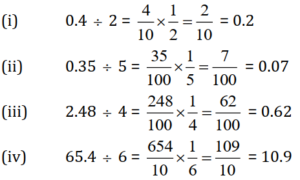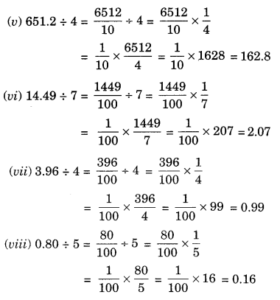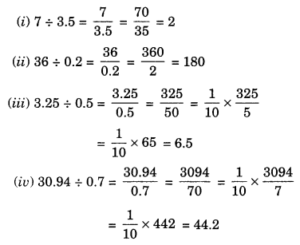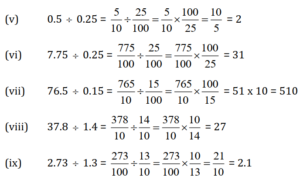# Free Ncert Solution for Class 7 Maths Chapter 2 Fractions and Decimals Exercise 2.5

Fractions and Decimals Class 7 Ex. 2.5;

New Ncert Solution for Class 7 Maths Chapter 2 Fractions and Decimals Free Solution;

Exercise 2.5

Question 1 :- Find:
(i) 0.4 ÷ 2
(ii) 0.35 ÷ 5
(iii) 2.48 ÷ 4
(iv) 65.4 ÷ 6
(v) 651.2 ÷ 4
(vi) 14.49 ÷ 7
(vii) 3.96 ÷ 4
(viii) 0.80 ÷ 5

Solution 1:-Question 2 :- Find:
(i) 4.8 ÷ 10
(ii) 52.5 ÷ 10
(iii) 0.7 ÷ 10
(iv) 33.1 ÷ 10
(v) 272.23 ÷ 10
(vi) 0.56 ÷ 10
(vii) 3.97 ÷10

Solution 2:-
(i) 4.8 ÷ 10 = 0.48             [Shifting the decimal point to the left by one place)
(ii) 52.5 ÷ 10 = 5.25          [Shifting the decimal point to the left by one place)
(iii) 0.7 ÷ 10 = 0.07           [Shifting the decimal point to the left by one place)
(iv) 33.1 ÷ 10 = 3.31         [Shifting the decimal point to the left by one place)
(v) 272.23 ÷ 10 = 27.223  [Shifting the decimal point to the left by one place)
(vi) 0.56 ÷ 10 = 0.056       [Shifting the decimal point to the left by one place)
(vii) 3.97 ÷ 10 = 0.397      [Shifting the decimal point to the left by one place)

Question 3 :- Find:
(i) 2.7 ÷ 100
(ii) 0.3 ÷ 100
(iii) 0.78 ÷ 100
(iv) 432.6 ÷ 100
(v) 23.6 ÷ 100
(vi) 98.53 ÷ 100

Solution 3:-

(i) 2.7 ÷ 100 = 0.027             [Shifting the decimal point to the left by 2 places)
(ii) 0.3 ÷ 100 = 0.003            [Shifting the decimal point to the left by 2 places)
(iii) 0.78 ÷ 100 = 0.0078       [Shifting the decimal point to the left by 2 places)
(iv) 432.6 ÷ 100 = 4.326       [Shifting the decimal point to the left by 2 places)
(v) 23.6 ÷ 100 = 0.236          [Shifting the decimal point to the left by 2 places)
(vi) 98.53 ÷ 100 = 0.9853     [Shifting the decimal point to the left by 2 places)

Question 4 :- Find :
(i) 7.9 ÷ 1000
(ii) 26.3 ÷ 1000
(iii) 38.53 ÷ 1000
(iv) 128.9 ÷ 1000
(v) 0.5 ÷ 1000

Solution 4:-
(i) 7.9 ÷ 1000 = 0.0079              [Shifting the decimal point to the left by 3 places)
(ii) 26.3 ÷ 1000 = 0.0263           [Shifting the decimal point to the left by 3 places)
(iii) 38.53 ÷ 1000 = 0.03853      [Shifting the decimal point to the left by 3 places)
(iv) 128.9 ÷ 1000 = 0.1289        [Shifting the decimal point to the left by 3 places)
(v) 0.5 ÷ 1000 = 0.0005             [Shifting the decimal point to the left by 3 places)

Question 5 :- Find :
(i) 7 ÷ 3.5
(ii) 36 ÷ 0 .2
(iii) 3.25 ÷ 0.5
(iv) 30.94 ÷ 0.7
(v) 0.5 ÷ 0.25
(vi) 7.75 ÷ 0.25
(vii) 76.5 ÷ 0.15
(viii) 37.8 ÷ 1.4
(ix) 2.73 ÷ 1.3

Solution 5:-Question 6 :- A vehicle covers a distance, 43.2-km in 2.4-litres of Petrol. How-much distance will it cover in one-litre of petrol ???

Solution 6:-
Since, 2.4-litres of petrol required to cover distance = 43.2-km;
So, 1-litre of petrol required to cover distance = 43.2/2.4 = 18-km;

Hence, the required distance = 18 km